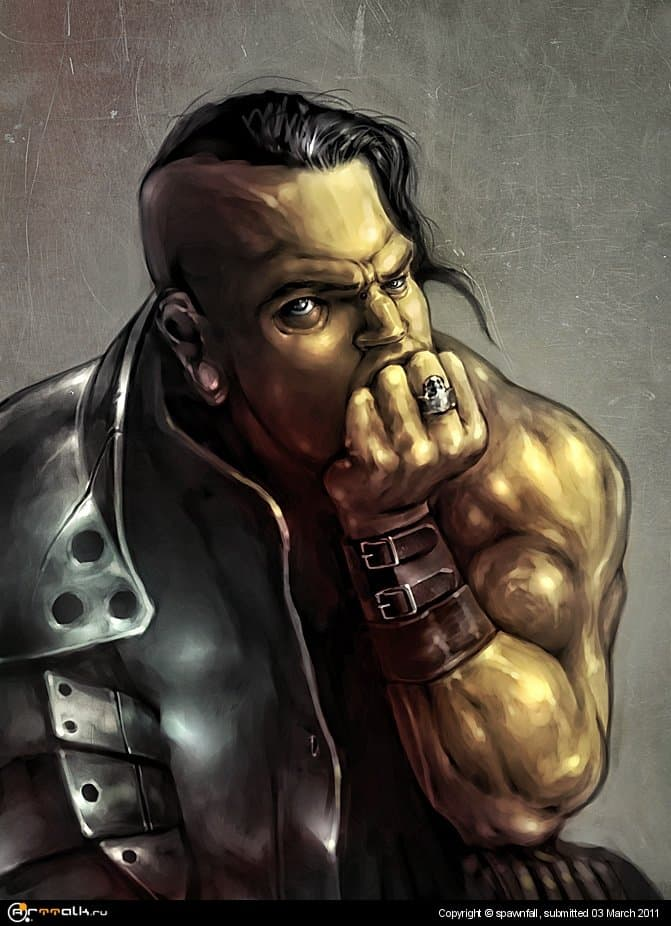Credit spawnfall

# орки любят панк

развлекаюсь

spawnfall

## Color Palette

давай, жду))))

Чёт очень сильно он кукурузку сжимает - аж вены повылазили на руках и на лбу - кукурузка с..ка резиновая)))))))))))))))))))))))))))))))))))))))))))))))))))))))))))))))))))

## Create an account

Register a new account

×

• Back
• Gallery

• #### Декоративно-прикладное искусство

• Sketchbooks
• Forums

• #### More

×
• Create New...